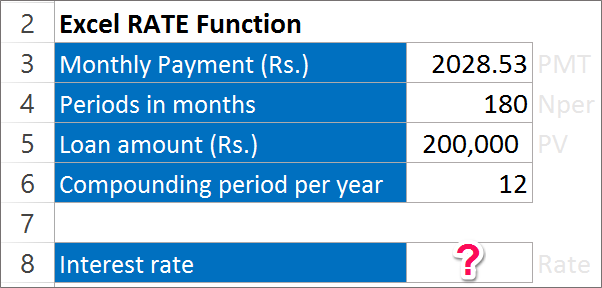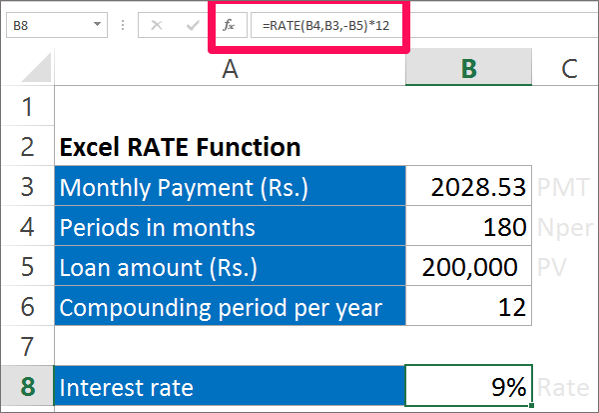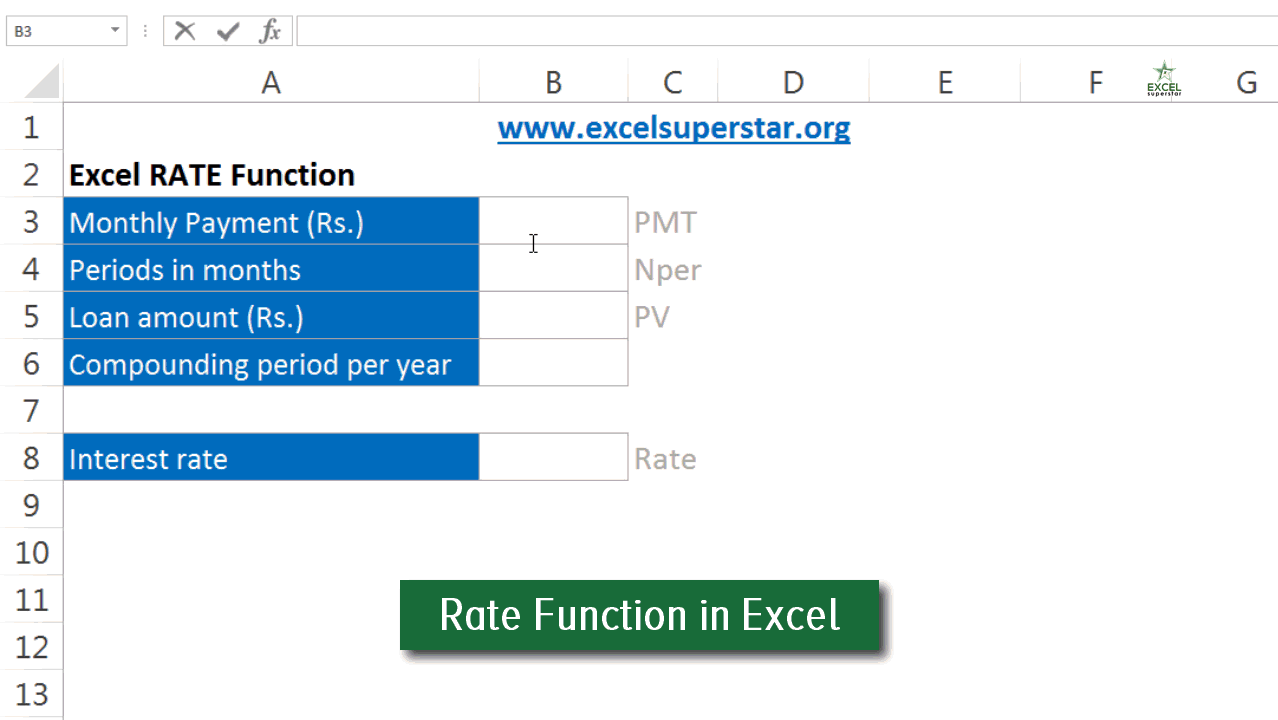Need Help? Chat with us

Rate Function | Rate formulas in Excel in Hindi

Rate Function – Excel Formulas & Function

The Rate function denotes the interest rate at which we need to pay our loan during a particular period of time. Let’s take a look on how can we use the Rate function to calculate the Interest rate. If you know the number of years, the amount of annuity payment and the Present value you can easily calculate the Rate function in Excel.

RATE(nper, pmt, pv, [fv], [type], [guess])

Special Offer for First Time Users

If you are the first time user and want to learn Microsoft office skills, then you can avail this special offer discount on your email.Where,

Rate stands for the rate at which the interest is provided.

Nper stands for the number of years or the time period of the loan.

PMT stands for the annuity payment.

PV stands for the present value.

FV stands for the future value. It is an optional argument.

Type stands for the when the payment is made. If not mentioned it is assumed that it is paid at the end of the period. It is an optional argument.

Guess stand for the assumption of what rate you think it should be. It is an optional argument. The Arguments in the Square brackets are optional.

Example of Rate Function

Calculate the annual interest rate that requires time period of 15 years, Rs. 2028.53 as monthly payment for a loan of 200,000.Solution:

Here, Nper = 180 as we need to make monthly payment so 15*12 will be 180

PMT = Rs. 2028.53

PV = Rs. 200,000

The other arguments in the box bracket are optional.You see the result as 9%

 Tip: The type in the arguments indicate when payment are due. If you enter 0 or omitted, it will assume at the end of the period and if you enter 1, it will assume at the beginning of the year. Rate function in ExcelRate function in Excel

Hope you like our tutorial on Rate Function for more cool and amazing trick of excel Like our Excel Superstar Facebook Page and subscribe to our Excel Superstar YouTube channel. Excel Superstar is the leading online training company, which provides Online Excel Course in Hindi. Connect with us and become an Excel Superstar.